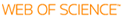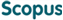### BROWSE

#### Related ResearcherYun, Aaram
Cryptography and Secure Computation Lab
Research Interests
• Cryptography, complexity theory, computer security

CRT-based fully homomorphic encryption over the integers

Cited 0 times inCited 0 times inTitle
CRT-based fully homomorphic encryption over the integers
Author
Issue Date
2015-07
Publisher
ELSEVIER SCIENCE INC
Citation
INFORMATION SCIENCES, v.310, pp.149 - 162
Abstract
In 1978, Rivest, Adleman and Dertouzos introduced the basic concept of privacy homomorphism that allows computation on encrypted data without decryption. It was an interesting work whose idea precedes the recent development of fully homomorphic encryption, although actual example schemes proposed in the paper are all susceptible to simple known-plaintext attacks. In this paper, we revisit one of their proposals, in particular the third scheme which is based on the Chinese Remainder Theorem and is ring homomorphic. It is known that only a single pair of known plaintext/ciphertext is needed to break this scheme. However, by exploiting the standard technique to insert an error to a message before encryption, we can cope with this problem. We present a secure modification of their proposal by showing that the proposed scheme is fully homomorphic and secure against the chosen plaintext attacks under the approximate GCD assumption and the sparse subset sum assumption when the message space is restricted to Z(2)(k). Interestingly, the proposed scheme can be regarded as a generalization of the DGHV scheme with larger plaintext space. Our scheme has (O) over tilde(lambda(5)) ciphertext expansion overhead while the DGHV has (O) over tilde(lambda(8)) for the security parameter lambda. When restricted to the homomorphic encryption scheme with depth of O(log lambda), the overhead is reduced to (O) over tilde(lambda). Our scheme can be used in applications requiring a large message space Z(Q) for log Q = (O) over tilde(lambda(4))or SIMD style operations on Z(Q)(k) for log Q = O(lambda), k = O(lambda(3)), with (O) over tilde(lambda(5)) ciphertext size as in the DGHV.
URI
https://scholarworks.unist.ac.kr/handle/201301/11532
URL
http://www.sciencedirect.com/science/article/pii/S002002551500184X#
DOI
10.1016/j.ins.2015.03.019
ISSN
0020-0255
Appears in Collections:
EE_Journal Papers
Files in This Item:
There are no files associated with this item.